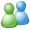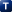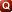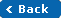Instant MessageSolution Library: Sub Topics: Questions## 20 Solution(s) found.

 1 2 3 4
Question:  Intuition to Econometrics
Question:  For the simple linear regression model, derive the estimates of the parameters. Using normal equations derive the estimates of constant and slope term
Question:  Derive the deviation form of beta 2 hat (deviation form of slope term)
Question:  Write a simple linear regression model in deviation form
Question:  Regression line passes through sample means of x and y
Question:  Show that the mean value of the estimated y is equal to the mean value of the actual y
Question:  Assuming a simple linear regression model, show that the residuals are uncorrelated with predicted y
Question:  What are deterministic and Statistical relationships? Distinguish between them.
Question:  Distinguish between regression, causation and correlation
Question:  Ordinary least squares
Question:  Why OLS? What is the main property of an OLS estimator?
Question:  Explain the first assumption of CLRM, i.e. 'Linearity of a model'
Question:  Explain the second assumption of CLRM, i.e. ' X values are assumed to be fixed in a repeated sampling'
Question:  Assumption 1: Model is linear in parameters
Question:  Assumption 2: x values are fixed in repeated sampling
Question:  Assumption 3: Error term has a zero mean
Question:  Assumption 4: 'Homoscedasticity'
Question:  Assumption 5:'No autocorrelation'
Question:  Assumption 6: 'No correlation between error term and x's'
Question:  Assumption 7: 'the number of observations n must be greater than the number of parameters to be estimated, k'
 Solution:
 1 2 3 4